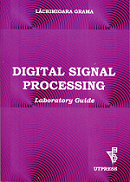## Digital Signal Processing - 3rd year AE

### Lecture

 Lecture Notes in DIGITAL SIGNAL PROCESSING - C. Rusu, L. Grama, RISOPRINT, Cluj-Napoca 2009 Lecture 1 Course Overview. Introduction to Digital Signal Processing Lecture 2 Discrete-Time Signals and Systems Lecture 3 Analysis of Discrete-Time Linear Time-Invariant Systems Lecture 4 Direct-Form Implementation of Discrete-Time Systems. Linear Time-Invariant Systems Characterized by Difference Equations Lecture 5 z-Transform Lecture 6 z-Domain Analysis of Linear Time-Invariant Systems. Fourier Series for Discrete-Time Periodic Signals Lecture 7 Fourier Transform for Discrete-Time Aperiodic Signals. Frequency Domain Characteristics of Linear Time-Invariant Systems Lecture 8 Discrete Fourier Transform Lecture 9 Fast Fourier Transform. Linear Time-Invariant Systems as Frequency Selective Filters Lecture 10 Linear-Phase Finite Impulse Response Filters. Design of Digital Finite Impulse Response Filters Lecture 11 Design of Digital Infinite Impulse Response Filters. Implementation of Discrete-Time Finite Impulse Response Systems Lecture 12 Implementation of Discrete-Time Infinite Impulse Response Systems Lecture 13 Quantization Lecture 14 Digital Signal Processing Summary. Exam Example

### Laboratory

DIGITAL SIGNAL PROCESSING -- laboratory guide - L. Grama, U.T.PRESS, Cluj-Napoca 2014

Introduction to MATLAB (Function for arithmetic mean)Discrete-Time Signals (Functions for unit impulse and unit step)

Sampling of Analog Signals. Correlation

Discrete-Time Linear Time-Invariant Systems

z-Transform and z-Domain Analysis of Linear Time-Invariant Systems

Laboratory 6
Test 1 - from laboratories 1, 2, 3, 4 and 5

Fourier Transform and Discrete Fourier Transform

Linear and Circular Convolution

Discrete-Time Linear Time-Invariant Systems as Frequency Selective Filters

Finite Impulse Response Filters. Design Methods

Infinite Impulse Response Filters. Indirect Design Methods

Laboratory 12
Test 2 - from laboratories 7, 8, 9, 10 and 11

Laboratory 13
Seminar - Implementation of Discrete-Time Systems

Laboratory 14
Seminar

### References

 Corneliu Rusu, Lăcrimioara Grama. Lecture Notes in Digital Signal Processing. Ed. Risoprint, 2009. John G. Proakis, Dimitris G. Manolakis. Digital Signal Processing: Principles, Algorithms and Applications. Pearson Education, 5th ed., 2022. Steven W. Smith. The Scientist and Engineer’s Guide to Digital Signal Processing. dspguide.com. Corneliu Rusu. Prelucrarea numerică a semnalelor. Ed. Risoprint, 2002. Corneliu Rusu. Prelucrări digitale de semnale. Ed. Mediamira, 2000. Lăcrimioara Grama. Digital signal processing: laboratory guide. Ed. U.T.Press, 2014. Sanjit K. Mitra. Digital signal processing: A Computer-Based Approach. McGraw-Hill international edition, 4th ed., 2011. MathWork. mathworks.com. Lăcrimioara Grama, Corneliu Rusu. Prelucrarea numerică a semnalelor - aplicații și probleme. Ed. U.T.Press, 2008. Lăcrimioara Grama, Alin Grama, Corneliu Rusu. Filtre numerice - aplicații și probleme. Ed. U.T.Press, 2008.

### Problems

 Solved problems Proposed problems